TitleBeginning Algebra
Answer/Discussion to Practice Problems
Tutorial 19: Practice Test on Tutorials 11 - 18

 Problems 1a - 1b:  Simplify the expressions.

 1a.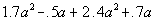Answer: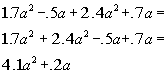1b.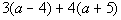Answer: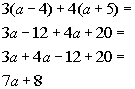Problem 2a:  Write the phrase as an algebraic expression and simplify if possible.

 2a.  The sum of 8 times a number and 4 subtracted from 16 times a number.Answer: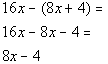Problems 3a - 3c:  Solve the given equation.

 3a.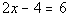Answer: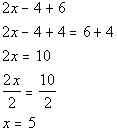3b.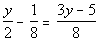Answer: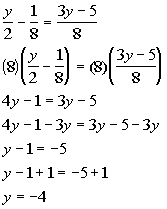3c.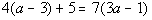Answer: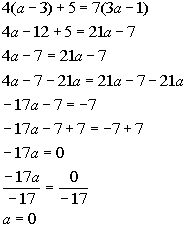Problems 4a - 4f:  Solve the following word problems.

 4a  The product of 5 and a number is 8 less than 7 times that number.Answer: Let x = the number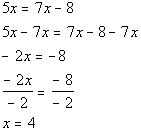The number is 4.

 4b.  Complimentary angles sum up to be 90 degrees.  Find the measure of each angle in the figure below.  Note that since the angles make up a right angle, they are  complementary to each other.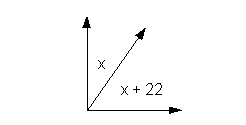Answer: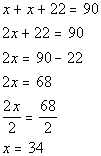The angles are 34 degrees and 56 degree.

 4c.   14.4 is what percent of 60?Answer: Let x = the percent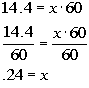x is 24%.

 4d.   You are wanting to buy a stereo system that costs \$325 plus tax.  If you are going to be taxed 8.25%, how much will be the final cost of the stereo system? (round to the nearest cent)Answer: Let x = final cost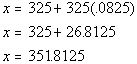The final cost would be \$351.81.

 4e.  You are traveling down I-40 at a constant speed of 70 mph.  How many hours will it take you to travel 385 miles?Answer: Let t = the number of hours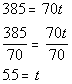It would take 5.5 hours.

 4f.   A total of \$1000 is deposited into two simple interest accounts.  On one account the annual simple interest rate is 5% and on the second account the annual simple interest rate is 7%.  The amount of  interest earned for 1 year was \$64.  How much is invested in each account?Answer: x = amount in 5% 1000 - x = amount in 7%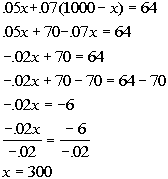Deposited \$300 at 5% and \$700 at 7%.

 Problems 5a - 5c:  Solve the inequality and graph the solution set.

 5a.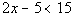Answer: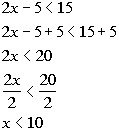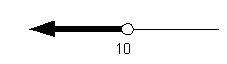5b.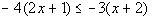Answer: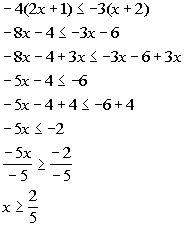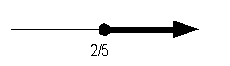5c.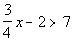Answer: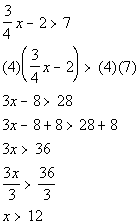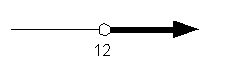Last revised on July 28, 2011 by Kim Seward.
All contents copyright (C) 2001 - 2010, WTAMU and Kim Seward. All rights reserved.